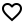Project Url: xizzhu/askIntroduction: Android SQLite Kotlin Extensions
More: Author   ReportBugs
Tags:

Kotlin extensions to simplify working with SQLite database.

• Gradle: Add the following to your `build.gradle`: ```gradle repositories { maven { url "https://jitpack.io" } }

``````
Usage
-----
### Create and Drop Tables
* To create a table, use the `createTable()` function:
```kotlin
database.createTable("tableName") {
it["textColumn"] = TEXT + PRIMARY_KEY
it["integerColumn"] = INTEGER + UNIQUE(ConflictClause.REPLACE)
it["anotherTextColumn"] = TEXT + FOREIGN_KEY("referenceTable", "referenceColumn")
}
``````

Each column must have one of the four types: `BLOB`, `INTEGER`, `REAL`, and `TEXT`. It can also also have one or more modifiers: `PRIMARY_KEY`, `NOT_NULL`, `UNIQUE`, `DEFAULT`, and `FOREIGN_KEY`.

• To delete a table, use the `dropTable()` function:

``````database.dropTable("tableName")
``````
• To check if a table exists, use the `hasTable()` function:

``````database.hasTable("tableName")
``````

### Create and Drop Indices

• To create an index, use the `createIndex()` function:

``````database.createIndex("indexName", "tableName", "column1", "column2")
``````
• To delete an index, use the `dropIndex()` function:

``````database.dropIndex("indexName")
``````

### Insert Values

To insert a row into a table, use the `insert()` function:

``````database.insert("tableName") {
it["textColumn"] = "random text"
it["integerColumn"] = 8964L
}
``````

### Update Values

To update an existing row, use the `update()` function:

``````database.update("tableName", { it["textColumn"] = "random new value" }) {
("integerColumn" eq 1L) and ("anotherTextColumn" eq "value")
}
``````

It supports simple conditions like `eq`, `less`, etc., and logical conditions like `and`, `or`, etc. The full list of supported conditions can be found here.

### Delete Values

• To delete all values from a table, use the `deleteAll()` function:

``````database.deleteAll("tableName")
``````
• To delete values matching certain conditions, use the `delete()` function:

``````database.delete("tableName") {
"integerColumn" eq 1L
}
``````

It supports same conditions as discussed in the Update Values section.

### Query Values

To query values from a table, use the `select()` function:

``````val query = database.select("tableName") {
"integerColumn" eq 1L
}
``````

It supports same conditions as discussed in the Update Values section.

The returned `Query` object can be further custmized by calling the `groupBy()`, `limit()` or other functions, e.g.:

``````query.groupBy("integerColumn")
.having { max("integerColumn") greater 1L }
``````

Note that the query is not executed, until `asCursor()` or one of the extension functions is called, e.g.:

``````// return a list of Pair<Integer, String>
query.toList { row ->
row.getInt("integerColumn") to row.getString("textColumn")
}

// return the Integer value of integerColumn in the first row
query.first { row ->
row.getInt("integerColumn")
}
``````

More about `Query` can be found here.

### Run a Transaction

• To run a transaction, use the `transaction()` function:

``````database.transaction {
}
``````

To abort the transaction, simply throw `TransactionAbortedException`.

• To run a transaction with a return value, use the `withTransaction()` function:

``````val value = database.withTransaction {
// your transaction code that returns a value
}
``````

``````Copyright (C) 2020 Xizhi Zhu

you may not use this file except in compliance with the License.
You may obtain a copy of the License at